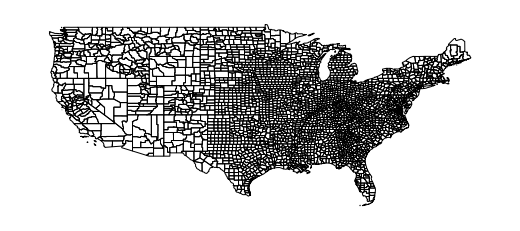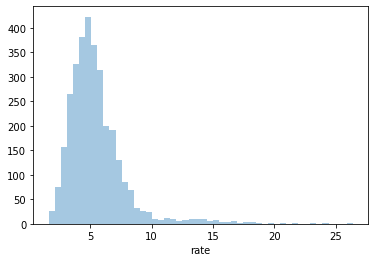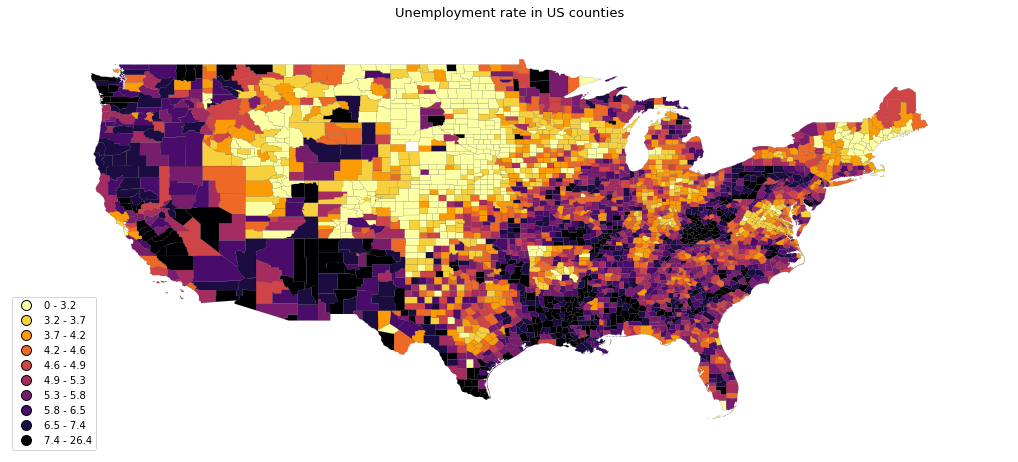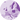# Choropleth map with python and geopandas

This post describes how to build a choropleth map with `python` and the `geopandas` library. It loads a `geojson` file of the US counties and draw the map with `geoplot`.

This post shows how to build a choropleth map for US counties. County border coordinates have been found here and stored on github here. Let's load this data stored at `geojson` format with `geopandas`, and draw a map with it thanks to `geoplot`.

``````# Import the geopandas and geoplot libraries
import geopandas as gpd
import geoplot as gplt

# Load the json file with county coordinates

# Make sure the "id" column is an integer
geoData.id = geoData.id.astype(str).astype(int)

# Remove Alaska, Hawaii and Puerto Rico.
stateToRemove = ['02', '15', '72']
geoData = geoData[~geoData.STATE.isin(stateToRemove)]

# Basic plot with just county outlines
gplt.polyplot(geoData, figsize=(20, 4));
``````Note: if you need more explanation on this step, there is this dedicated post. More generally, have a look to the background map section of the python graph gallery.

A choropleth map shows the distribution of a numeric variable on each items of a map. Let's consider the unemployment rate of each US county. Data comes from the Bureau of Labor Statistics, found here and stored here.

``````# library
import pandas as pd
import seaborn as sns

# Show the distribution of unemployment rate
sns.distplot( data["rate"], hist=True, kde=False, rug=False );
``````## Merge spatial & numeric data

Both source of information need to be merged in order to be represented on the same figure. This can be done thanks to the `merge()` function.

``````fullData = geoData.merge(data, left_on=['id'], right_on=['id'])
id GEO_ID STATE COUNTY NAME LSAD CENSUSAREA geometry state county rate
0 1001 0500000US01001 01 001 Autauga County 594.436 POLYGON ((-86.49677 32.34444, -86.71790 32.402... Alabama Autauga County 5.1
1 1009 0500000US01009 01 009 Blount County 644.776 POLYGON ((-86.57780 33.76532, -86.75914 33.840... Alabama Blount County 5.1

## Choropleth map with `GeoPlot`

The hardest part of the job has been done. Now, the `choropleth()` function of the `geoplot` library allows to build the choropleth pretty easily. The `hue` parameter expects the name of the column we want to use to control the color of each county.

The hardest part is probably to pick the right type of color palette. `Geoplot` comes with both continuous and categorical binning schemes, i.e. methods that split a sequence of observations into some number of bins.

``````# Initialize the figure
import matplotlib.pyplot as plt
fig, ax = plt.subplots(1, 1, figsize=(16, 12))

# Set up the color sheme:
import mapclassify as mc
scheme = mc.Quantiles(fullData['rate'], k=10)

# Map
gplt.choropleth(fullData,
hue="rate",
linewidth=.1,
scheme=scheme, cmap='inferno_r',
legend=True,
edgecolor='black',
ax=ax
);

ax.set_title('Unemployment rate in US counties', fontsize=13);``````## Contact & Edit

👋 This document is a work by Yan Holtz. Any feedback is highly encouraged. You can fill an issue on Github, drop me a message onTwitter, or send an email pasting `yan.holtz.data` with `gmail.com`.